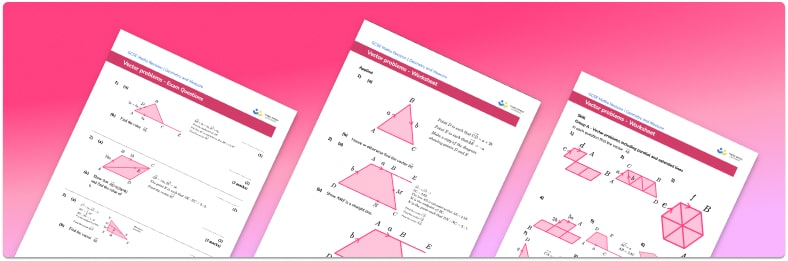# Vector Problems Worksheet• Section 1 of the vector problems worksheet contains 36 skills-based vector problems questions, in 3 groups to support differentiation
• Section 2 contains 4 applied vector problems questions with a mix of worded problems and deeper problem solving questions
• Section 3 contains 3 foundation and higher level GCSE exam style vector problems questions
• Answers and a mark scheme for all vector problems questions are provided
• Questions follow variation theory with plenty of opportunities for students to work independently at their own level
• All questions created by fully qualified expert secondary maths teachers
• Suitable for GCSE maths revision for AQA, OCR and Edexcel exam boards

• This field is for validation purposes and should be left unchanged.

You can unsubscribe at any time (each email we send will contain an easy way to unsubscribe). To find out more about how we use your data, see our privacy policy.

### Vector problems at a glance

A vector describes a movement and has both a magnitude (a size or length) and a direction. The vector describing the movement from A to B can be denoted in three different ways: a capital AB with an arrow pointing to the right above the two letters, a, or a. The vector that is a movement in the opposite direction, from B to A, is written as BA with an arrow pointing to the right above the two letters, –a, or –a

Vectors can also be described using a column vector notation. The top number of a column vector is the x component and tells us the movement parallel to the x-axis (left or right) and the bottom number is the y component which tells us the movement parallel to the y-axis (up or down). For example, the vector of 5 on top of -3 in large round brackets describes the movement of 5 to the right, and 3 down.

A scalar is a quantity that has a magnitude (a size) but no direction. Vectors can be multiplied by scalar quantities to make them shorter, longer or change their direction. For example, the vector 2a is twice the magnitude of the vector a.

Two vectors are parallel if they have the same direction. For example, in the parallelogram ABCD, the vector that describes the movement from A to B can be written as a. We know that the sides AB and DC in a parallelogram have the same direction, so they are parallel. Also, the length of D to C is equal to the length of A to B, so DC can also be labelled with the vector a

We can use vector addition and subtraction to combine vectors as a way of finding a pathway from one point to another. For example, if we move along the direction of the vector a and then along the vector b, we can describe the direction of the resultant vector to be a+b. The shortest distance between the two points A and B is known as the displacement vector.

Solving problems with vectors may involve travelling part way along line segments, for example to the midpoint of a line. This can be done using scalar multiplication as, for this example, we would halve the length of the vector to reach the midpoint. The vector sum of a number of different vectors or multiples of vectors may need to be found in order to create a valid path from the starting point to the end point of a problem.

The magnitude of a vector is the length or size of the vector. This can be found by drawing a right triangle illustrating the x and y components of a vector as the base and the height of the triangle. The vector itself is the hypotenuse of the triangle. The magnitude of the vector can be determined using Pythagoras’ Theorem to calculate the length of the hypotenuse.

A unit vector is a vector with magnitude 1. For example, the vector of 1 on top of 0 in large round brackets is a movement of 1 in the x direction (due east). Using Pythagoras’ Theorem, the magnitude of the vector is the square root of 12+02 which is equal to 1. As the magnitude of a vector is a length, the value is always positive (you cannot have a negative length). Calculating the magnitude and direction of a vector is explored further at A level along with the resultant force applied to an object.

Looking forward, students can then progress to additional geometry worksheets, for example the Pythagoras’ Theorem worksheet, the transformations worksheet, or the forming and solving equations worksheet.For more teaching and learning support on geometry our GCSE maths lessons provide step by step support for all GCSE maths concepts.

## Do you have KS4 students who need more focused attention to succeed at GCSE?There will be students in your class who require individual attention to help them succeed in their maths GCSEs. In a class of 30, it’s not always easy to provide.

Help your students feel confident with exam-style questions and the strategies they’ll need to answer them correctly with our dedicated GCSE maths revision programme.

Lessons are selected to provide support where each student needs it most, and specially-trained GCSE maths tutors adapt the pitch and pace of each lesson. This ensures a personalised revision programme that raises grades and boosts confidence.

Find out more